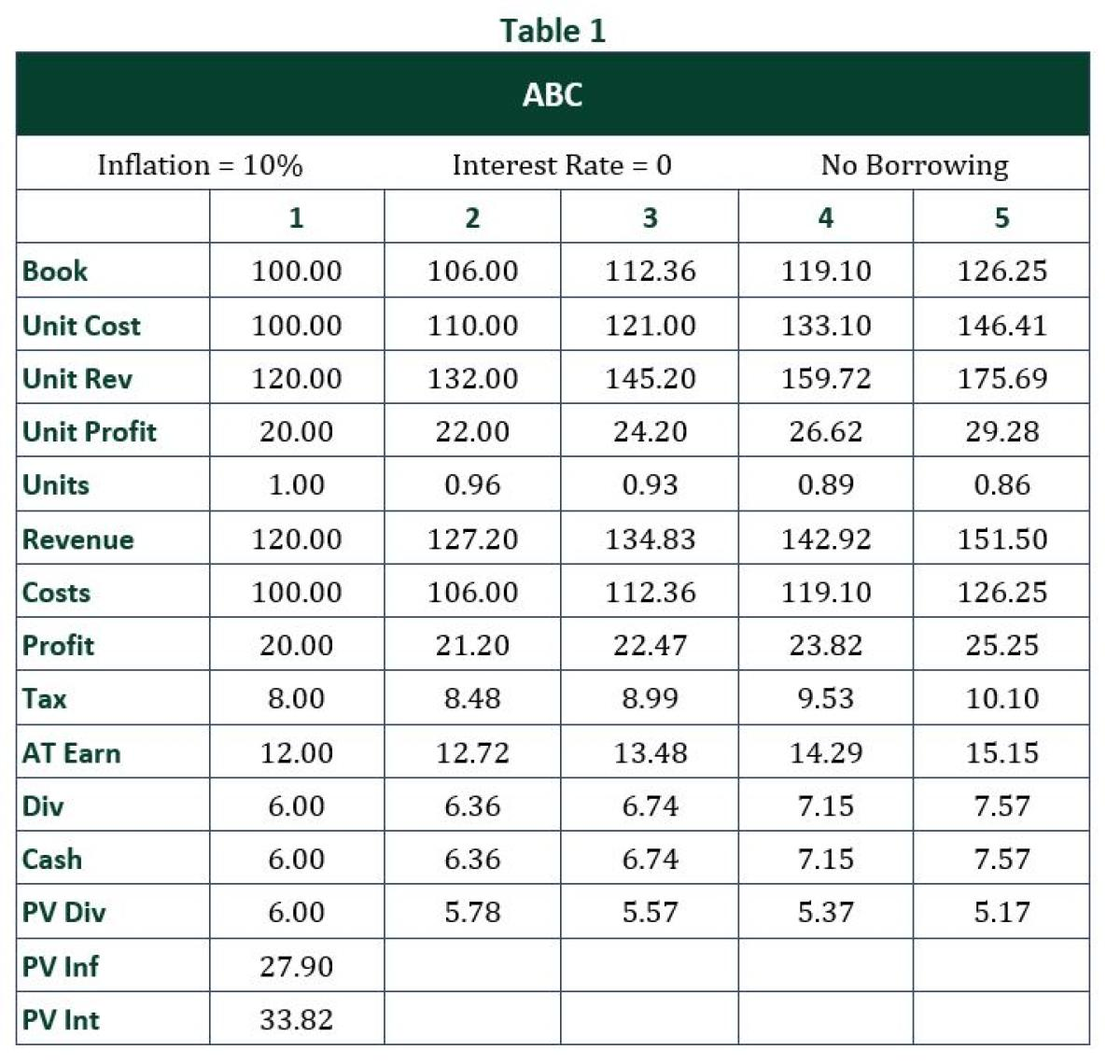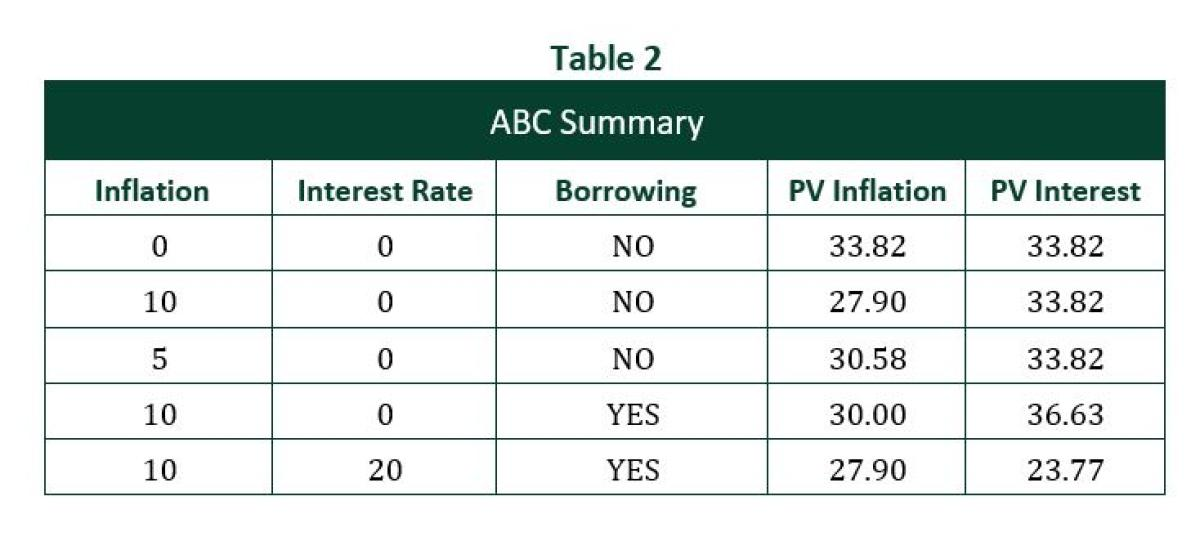Back to Portfolio for the Future™

# Inflation, Interest Rates and Equity Evaluation

February 1, 2023

By Dan diBartolomeo, President and founder of Northfield Information Services, Inc. Based in Boston since 1986, Northfield develops quantitative models of financial markets.

Introduction

Equity markets have recently seen significant volatility associated with spikes in inflation across developed countries (e.g., US and UK), and the associated expectations of ongoing interest rate increases by central banks. Energy cost increases arising out of sanctions on the Russia invasion of Ukraine have convinced some investors that broad inflation may extend well beyond the post-COVID recovery.

In this article we will explore the dependence of equity prices on inflation and interest rates in two ways. First, we will consider the impact of various combinations of interest rates and inflation on the financial statements of two hypothetical companies. We will then extend these results into a Gordon style dividend discount model for theoretical confirmation that real interest rates should be a dominant factor, rather than nominal interest rates or inflation considered separately. The final portion of the article will present a proprietary method for estimating real growth of companies over time.

A key finding of this work is that we assert an important departure from the assumptions of the Modigliani-Miller Theorem (American Economic Review, 1958). This theory argued that the value of an enterprise could be defined solely as the present value of future earnings and was unrelated to capital structure if markets are efficient. The theory was later revised to include the tax deductibility of interest payments (American Economic Review, 1963). It is our assertion that taxation, inflation, the availability of credit, and interest rates all interact to impact enterprise valuation.

A Company Level Hypothetical

We will begin with looking at a hypothetical company ABC under five different economic scenarios with various combinations of inflation, interest rates, and restrictions on borrowing. The “fundamentals” of ABC are constant:

1. Unit cost of goods sold starts at 100

2. Unit revenue starts at 120

3. Tax rate is 40%

4. Dividends are 50% of after-tax earnings

We simulated five years of activity under each scenario and report the present value of the next five years of dividends under two scenarios (using inflation as the discount rate and using the interest rate as the discount rate).

In Table 1 we present the simulated financial statement of ABC assuming no borrowing is allowed:We then created a similar analysis for ABC across multiple scenarios for inflation, interest rates, and availability of borrowing (Table 2). As taxes are levied on nominal profits not adjusted for inflation, firms restricted from borrowing will be unable to finance even level unit operations. When both taxes and inflation are present, but borrowing is allowed, the extreme values of the real interest rate have a significant impact on valuation. For “slow process” enterprises (e.g., a wine maker who must age the product for years before sale) the impact of taxation not being adjusted for inflation is even more profound.Valuation in Theory

We begin with the Gordon Dividend Discount Model, the simplest “textbook” approach for valuing companies. It was first proposed in Gordon and Shapiro (Management Science, 1956) and was extended in Gordon (Review of Economics and Statistics, 1959). The model assumes companies survive in perpetuity and that cost of capital and company growth rate is constant over time.

P = D / (k – g)

P is the value of a share of stock

D is the current dividend

k is the cost of capital

g is the assumed perpetual growth rate

We can rewrite the Gordon model to isolate desired features.

P=D / (k – g) = D / [ (Rp + I) – (Gr+F)] = D / [ Rp + Ir + F – Gr - F]

P = D / [ (Rp + Ir) – Gr]

Rp is the annual risk premium

I is the nominal interest rate

Ir is the real interest rate

Gr is the real growth rate

F is the inflation rate

It should be clear that Inflation (F) drops out, leading to a form where the important inputs are the equity risk premium (Rp), the real interest rate (Ir) and the assumed real growth rate Gr. While beyond the scope of this article, our views on equity risk premia going forward appeared in diBartolomeo and Kantos (Journal of Asset Management, December 2020).

One key aspect of the specification is that we have not defined a time horizon for the real interest rate. Is the real interest rate short term, Treasury Bill rates versus recent CPI) or should we be thinking about longer horizons: 5 years, 10 years, 30 years? While companies do not survive in perpetuity, equity valuations imply average “half-lives” over twenty years. See diBartolomeo (Journal of Investing, 2010).

Leibowitz (Financial Analyst Journal, September 1989) recognized the offsetting influence on inflation on corporate profits, effectively measuring “equity duration” against real interest rates. Equity durations against nominal interest rates looks short (e.g., a few years) because the volatility of real interest rates is much lower than the volatility for nominal rates. Equity durations are long and consistent with theory when measured against changes in real interest rates.

Rubinstein (Journal of Finance, 1976) provides an investor utility model that focuses on the present value of future consumption expenditure similar to the way a DDM represents investment earnings. If investors care about funding consumption in the far future, they should value economic payouts on similar horizons. Muijsson, Fishwick and Satchell (Economics, 2015) assert that the relative outperformance of low beta, low volatility strategies was related to pervasive declines in interest rates. We more precisely ascribe this differential effect to the fact that interest rates are much more volatile for short maturities, impacting near term cash flows more. See Fixing Active Management: Why Value Investing Works (or At Least Has Worked)! (northinfo.com).

Estimating Real Growth Rates with the EUPS Model

While economists spend a lot of time forecasting real growth from a macro perspective, estimating growth in individual firm profits has been the domain of fundamental analysts. Back in 1989, Northfield invented a model called EUPS to estimate what we then called long-term “true growth.” The EUPS model says that a firm grows by finding projects to perform where the likely return on capital exceeds the cost of that capital. Both finding high return projects and lowering the cost of capital provide new opportunities to grow.

Imagine we start a company S that does nothing but hold cash in an interest-bearing account. Due to the compounding of interest, the reported earnings of S will get larger year by year, but this isn’t doesn’t fit with our common understanding of the growth of a business.

To illustrate the EUPS idea, let’s take a typical company M that has annual earnings of \$10 per share, pays a \$4 dividend and retains \$6 in earnings. Now let’s recast the transaction. Assume the company earns \$10 per share, pays out a \$10 dividend and on the dividend pay-out date holds an offering of new shares to existing shareholders at the market price of the stock. If we limit the number of new shares offered such that out of the \$10 dividend just received, each investor invests \$6 back into new shares. From a cash flow perspective, nothing has changed. The firm earned \$10, the net dividend paid was \$4 and the firm retained \$6.

What is different is that the shares-outstanding has increased. We are inferring the cost of capital for retained earnings by making the transaction an explicit issuance of new equity. By calculating earnings per share with the adjusted value for shares outstanding we get a much better estimate of true growth of the enterprise.

The growth rate of EUPS (we call this “true” growth) will be positive if the marginal return on equity is greater than the cost of marginal equity. Conversely, growth of EUPS will be negative if the marginal cost of capital is greater than the marginal return on equity. The classical definition of a free competitive market would suggest that the marginal return on capital should be driven to the cost of that capital, so the relative rate of true growth at the firm level should converge on zero. In the long run, the expected real growth rate (as measured by EUPS) of any firm should regress to the overall real growth rate of the total economy over time.

Looking Back and Looking Forward

If we believe that equity valuation should be assessed against expectations of the future, we can estimate the “breakeven inflation rate” that would equate yields from fixed coupon US Treasury Bonds and TIPS (inflation protected). The ten-year breakeven inflation rate is presented in the chart below from the St. Louis Federal Reserve. As you can see, the implied inflation rate has been generally just over 2% with two short-lived dips during the Global Financial Crisis and the height of the COVID pandemic. These expectations are also consistent with realized inflation over the past ten years at an annual geometric mean rate of 2.41%. A similar analysis of the five-year breakeven inflation yields an extremely similar result, against a five-year realized inflation of 2.61%.

The striking part of this data is that despite the heavy coverage of inflationary pressures in both the general and financial press, the inference one can draw from actual market data is that investors expect long-term inflation to be only marginally higher than the typical central bank target of 2% annually.

Northfield research